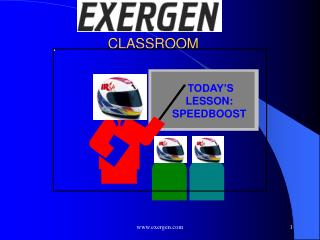DownloadDownload PresentationCLASSROOM

CLASSROOM

Download PresentationCLASSROOM

- - - - - - - - - - - - - - - - - - - - - - - - - - - E N D - - - - - - - - - - - - - - - - - - - - - - - - - - -
Presentation Transcript

1. CLASSROOM TODAY’S LESSON: SPEEDBOOST www.exergen.com

2. Faster Start-up Conventional Control 8 hr • Direct Control of Heater Surfaces and Product Temperature Reduces Adjustments Required IR Control 1 hr www.exergen.com

3. Reduced Scrap Conventional Control \$1000 per set-up • Less Product is Scrapped for Adjustments IR Control \$100 per set-up www.exergen.com

4. Increasing Speeds via Non-Invasive IR-Monitored Heat Balance www.exergen.com

5. Frontiers • Principles of the Heat Balance in Time and Space • The Speed Boost Equation • Balanced Heat Input via IR Control • Applications • Laminating, Drying, Printing, Heat Sealing, Color Copying • High Speed Event Detection www.exergen.com

6. Principles of the IRt/c: With Heat Balance • Automatically Computes Heat Balance, Using Material Properties Alone • Can be Configured for Unpowered or Powered Configurations www.exergen.com

7. T T T T f w s a q Radiation + R R R f t o Convection Heat Transfer R  R  R f t o   T  T  T  T f s a a R o Non-Invasive Fluid Temperature in Tubing via IRt/c Heat Balance www.exergen.com

8. Thermal Energy Balance in Space and Time:The Time Domain www.exergen.com

9. Jean Baptiste Joseph Fourier1768-1830 • Fourier’s Equation of Heat Conduction • Unsteady State Heat Conduction for Moving Materials www.exergen.com

10. Pierre Simon Marquis de LaPlace1749 -1827 • Laplace Transform Method of Solution • Converts Partial Differential Equation to Ordinary Differential Equation www.exergen.com

11. Francesco Pompei1948 - • New Method of Solution Leads to a General Equation for Non-Contact Temperature Monitoring of Internal Temperatures of Moving Materials www.exergen.com

12. Which simplifies to www.exergen.com

13. Deriving The Speed Boost Equation • Set the surface temperature equal to the center temperature, then the equation reduces to • Since K2/K1 is a function only of material properties and speed: www.exergen.com

14. The Speed Boost Equation The ratio can be formed, which then becomes: • General Equation for Non-Contact IR Temperature Monitoring of Internal Temperatures of Moving Materials is Combined with Surface Temperature • Leads to Uniform Material Temperature When Controlled via the Speed Boost Equation • Which Forces the Control System to Apply Heat at an Optimally Balanced Rate www.exergen.com

15. Applying The Speed Boost Equation www.exergen.com

16. Speed Boost Equation is Generally Linear for Most Applications 50 % Increase 25 25 50 Speed % Increase www.exergen.com

17. Implementing Speed Boost to Include Non-Linearities • Apply step-wise speed increases in accordance with speed boost equation, and renormalize at new operating condition to account for property changes. • For variable speed systems, program to follow the characteristic curve. Speed Changes Followed by Renormalization % Increase 50 25 25 50 Speed % Increase www.exergen.com

18. Too To Ts Example Speed Boost: Laminating • Existing Set-up: Too = 105 C Ts = 85 C To = 25C • New Set-up: Too = 120 C Ts = 85 C To = 25C • Potential Speed Increase*: • 25% *Assuming all other factors are permitting www.exergen.com

19. Too Ts To Example Speed Boost: Drying • Existing Set-up: • Too = 260 C • Ts = 85 C • To = 25 C • New Set-up: • Too = 260 C • Ts = 85 C • To = 40 C (with preheat) • Potential Speed Increase*: • 33% *Assuming all other factors are permitting www.exergen.com

20. Precision Drying Control for Maximum Production Speed Relative Temperatures at IRt/c Locations Dry-Out Point (Phase Change) www.exergen.com

21. To Too Ts Example Speed Boost: Heat Sealing • Existing Set-up: Too = 150 C Ts = 120 C To = 25 C • New Set-up: Too = 150 C Ts = 120 C To = 45 C (with preheat added) • Potential Speed Increase: • 27% www.exergen.com

22. Too Ts To Example: High Speed Color Copy Process Paper Flow Energy Flow www.exergen.com

23. To IRt/c. SV Ts Ts Tw Overcoming Thermal Delays due to Mass of Rollers www.exergen.com

24. Speed Boost Equation • Above Can Be a Simplified Control Algorithm • Keep Equation Balanced to Within a Few % to Avoid Non-Uniformity in Material Temperature Product Surface - setpoint Heat Source Temperature Product Input Control Loop Gain www.exergen.com

25. Too To Ts Example Speed Boost: Laminating • Existing Set-up: Too = 105 C Ts = 85 C To = 25C • New Set-up: Too = 120 C Ts = 85 C To = 25C • Potential Speed Increase*: • 25% *Assuming all other factors are permitting www.exergen.com

26. Too Ts To Example Speed Boost: Drying • Existing Set-up: • Too = 260 C • Ts = 85 C • To = 25 C • New Set-up: • Too = 260 C • Ts = 85 C • To = 40 C (with preheat) • Potential Speed Increase*: • 33% *Assuming all other factors are permitting www.exergen.com

27. To Too Ts Example Speed Boost: Heat Sealing • Existing Set-up: Too = 150 C Ts = 120 C To = 25 C • New Set-up: Too = 150 C Ts = 120 C To = 45 C (with preheat added) • Potential Speed Increase: • 27% www.exergen.com

28. You Cannot Know For Sure That the Product is Right Unless You Look...WithEXERGENIRSensors www.exergen.com